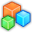# Microsoft Small BasicProgram Listing: HFR208-0
' Another simple analog clock
' Original Author : Chris Taylor
' Updated By : BKB

' User Customizations
bgColor = "Silver"
clockTickColor = "Black"
secondHandColor = "Red"
minuteHandColor = "#222222"
hourHandColor = "Black"
clockSize = 200

' Initialize the Graphics Window
GraphicsWindow.Hide()
GraphicsWindow.Title = "Analog Clock"
GraphicsWindow.Width = clockSize
GraphicsWindow.Height = clockSize
GraphicsWindow.Top = 0
GraphicsWindow.Left = Desktop.Width - clockSize-6
GraphicsWindow.BackgroundColor = bgColor
GraphicsWindow.CanResize = "False"

halfSize = clockSize/2

' Set up a timer to update the clock every 10th of a second
Timer.Interval = 100
Timer.Tick = OnTick

DrawClockFace()
GraphicsWindow.Show()

' SubRoutines...
'========================================
' Tick routine called every 10th of a second to update the clock display
Sub OnTick
' Clear the current clock hands
GraphicsWindow.BrushColor = bgColor
GraphicsWindow.FillEllipse(12,12,clockSize-25,clockSize-25)

DrawHourHand()
DrawMinuteHand()
DrawSecondHand()
EndSub
'========================================
Sub DrawSecondHand
value = Clock.Second + Clock.Millisecond/1000
period = 60
length = halfSize-15
width = 1
GraphicsWindow.PenColor = secondHandColor
DrawHand()
EndSub
'========================================
Sub DrawMinuteHand
value = Clock.Minute + Clock.Second/60
period = 60
length = 7*(halfSize-15)/8 '-20
width = 2
GraphicsWindow.PenColor = minuteHandColor
DrawHand()
EndSub
'========================================
Sub DrawHourHand
value = Clock.Hour + Clock.Minute/60
period = 12
length = 3*(halfSize-15)/4
width = 3
GraphicsWindow.PenColor = hourHandColor
DrawHand()
EndSub
'========================================
' DrawHand - draws the clock hand based on the values of the following global variables
' value - The value used to calculate the angle of the line
' period - The number that represents a full 360 degree rotation.
' For the minute and second hands this is 60, for the hour hand this is 12
' length - The length of the line to be drawn
' width - The width of the pen used to draw the line
Sub DrawHand
GraphicsWindow.PenWidth = width
angle = Math.Pi*(4*value-period)/(2*period)
tx = halfSize+length*Math.Cos(angle)
ty = halfSize+length*Math.Sin(angle)
GraphicsWindow.DrawLine(halfSize, halfSize, tx, ty)
EndSub
'========================================
' DrawClockFace - draws the tick marks around the clock face.
Sub DrawClockFace
GraphicsWindow.PenColor = clockTickColor
For t = 0 To 29
angle = (Math.Pi*t / 30)
cos = Math.Cos(angle)
sin = Math.Sin(angle)

If Math.Remainder(t, 5) = 0 then
GraphicsWindow.PenWidth = 3
tickLength = clockSize-2
Else
GraphicsWindow.PenWidth = 1
tickLength = clockSize-10
EndIf
tx = halfSize + cos*(tickLength/2)
ty = halfSize + sin*(tickLength/2)
GraphicsWindow.DrawLine(tx, ty, tx-cos*tickLength, ty-sin*tickLength)
EndFor
GraphicsWindow.BrushColor = bgColor
GraphicsWindow.FillEllipse(15,15,clockSize-30,clockSize-30)
EndSub
'========================================